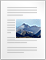Home » Physics » Mathematics revision sheet for class 11 and class 12 physics

# Mathematics revision sheet for class 11 and class 12 physics

[latexpage]

Here are the Mathematics revision sheets for class 11 and class 12 physics

## Differentiation

We have two quantities x and y such that $y=f(x)$ where $f(x)$ is some function of x. We may be interested in finding the followings things

1. $\frac{dy}{dx}$

2. Maximum and Minimum values of y.It can be found with the method of Maxima and Minima

$\frac{dy}{dx}$ is the called the derivative of y w.r.t to x

It is defined as

$\frac{dy}{dx}=\lim_{\Delta x \to 0}\left ( \frac{\Delta y}{\Delta x} \right )$

Some commonly known functions and their derivatives are:-

Some important and useful rules for finding derivatives of composite functions

1. $\frac{d}{dx}(cy)=c\frac{dy}{dx}$ where c is constant
2. $\frac{d}{dx}(a+b)=\frac{da}{dx} + \frac{da}{dx}$ where a and b are function of x
3. $\frac{d}{dx}(ab)=a\frac{db}{dx}+b\frac{da}{dx}$
4. $\frac{d}{dx}(\frac{a}{b})=\frac{[b\frac{da}{dx}-a\frac{db}{dx}]}{b^{2}}$
5. $\frac{dy}{dx}=(\frac{dy}{da})(\frac{da}{dx})$
6. $\frac{d^{2}y}{dx^{2}}=(\frac{d}{dx})(\frac{dy}{dx})$

Maximum and Minimum values of y

Step 1:
fine the derivative of y w.r.t x

$(\frac{dy}{dx})$

Step2:
Equate

$\frac{dy}{dx}=0$

Solve the equation to find out the values of x

Step3:
find the second derivative of y w.r.t x and calculate the values of

$\frac{d^{2}y}{dx^{2}}$

for the values of x from step2

if $\frac{d^{2}y}{dx^{2}}>0$ then the value of x corresponds to mimina of y then $y_{min}$ can be find out by putting this value of x

if $\frac{d^{2}y}{dx^{2}}<0$ then the value of x corresponds to maxima of y then $y_{max}$ can be find out by putting this value of x

## Integration

$I=\int_{a}^{b}f(x)dx$

It reads as integration of function f(x) w.r.t. x within the limits from x=a to x=b.

Integration of some important functions are

$\int sinx dx=-cosx$

$\int cosx dx=sinx$

$\int sec^{x}dx=tanx$

$\int cosec^{x}dx=-cotx$

$\int \frac{1}{x}dx=lnx$

$\int x^{n}dx=\frac{x^{n+1}}{n+1}$

$\int e^x dx=e^x$

Useful rules for integration are

$\int cf(x)dx=c\int f(x)dx$

$\int[f(x)+h(x)]=\int f(x)dx+\int h(x)dx$

$\int f(x)g(x)dx=f(x)\int g(x)dx -\int\left ( f'(x)\int g(x)dx \right ) dx$

## Trigonometry

Properties of trigonometric functions

1. Pythagorean identity

$sin^2 A +cos^2 A=1$

$1+tan^ A=sec^2 A$

$1+cot^2 A=cosec^2 A$

2. Periodic function

$sin(A+2\pi)=sinA$

$cos(A+2\pi)=cosA$

3.Even-Odd Identities

$cos(-A)=cos(A)$

$sin(-A)=-sin(A)$

$tan(-A)=-tan(A)$

$cosec(-A)=-cosec(A)$

$sec(-A)=sec(A)$

$cot(-A)=-cot(A)$

4. Quotient identities

$tan(A)=\frac {sin A}{cos A}$
$cot(A)=\frac{cos A}{sin A}$

5. Co-function identities
$sin\left ( \frac{\pi}{2}-A \right )=cos(A)$

$cos\left ( \frac{\pi}{2}-A \right )=sin(A)$

$tan \left ( \frac{\pi}{2}-A \right )=cot(A)$

$cosec \left( \frac{\pi}{2}-A \right )=sec(A)$

$sec\left ( \frac{\pi}{2}-A \right )=cosec(A)$

$cot\left ( \frac{\pi}{2}-A \right )=tan(A)$

6. Sum difference formulas
$sin(A\pm B)=sin(A)cos(B) \pm sin(B)cos(A)$

$cos(A \pm B)=cos(A)cos(B) \mp sin(A)sin(B)$

$tan(A \pm B)=\frac {tan(A) \pm tan(B)}{1 \mp tan(A) tan (B)}$

7. Double angle formulas
$cos(2A)=cos^2(A)-sin^2(A)=2cos^2 (A)-1=1-2sin^2(A)$

$sin(2A)=2sin(A)cos(A)$
$tan(2A)=\frac{2tan(A)}{1-tan^2(A)}$

8. Product to sum formulas

$sin(A)cos(B)=\frac {1}{2}[cos(A-B)-cos[A+B]$

$cos(A)cos(B)=\frac {1}{2}[cos(A-B)+cos[A+B]$

$sin(A)cos(B)=\frac {1}{2}[sin(A+B)+sin[A-B]$

$cos(A)sin(B)=\frac {1}{2}[sin(A+B)-sin[A-B]$

9. Power reducing formulas
$sin^2 A=\frac{1-cos(2A)}{2}$

$cos^2 A=\frac{1+cos(2A)}{2}$

$tan^2 A=\frac{1-cos(2A)}{1+cos(2A)}$

10. reciprocal identities

$Sin(A)=\frac{1}{cosec(A)}$

$cos(A)=\frac{1}{sec(A)}$

$tan(A)=\frac{1}{cot(A)}$

$cosec(A)=\frac{1}{sin(A)}$

$Sec(A)=\frac{1}{cos(A)}$

$cot(A)=\frac{1}{tan(A)}$

## Binomial Theorem

$(a+b)^n=C_{0}^{n}a^{n}+C_{1}^{n}a^{n-1}b+C_{2}^{n}a^{n-2}b^2+………+C_{n}^{n}b^{n}$
From the binomial formula, if we let a = 1 and b = x, we can also obtain the binomial series which is valid for any real number n if |x| < 1.
(1+x)^n=1+nx+\frac{n(n-1)}{2!}x^2+\frac{n(n-1)(n-2)}{3!}x^3+………..

## Geometric Series

$a,aq,aq^2,aq^3,aq^4………..aq^{n-1}$ where q is not equal to 0, q is the common ratio and a is a scale factor.The formula for the sum of the first n numbers of geometric progression
$S_{n}=\frac{a(1-q^n)}{(1-q)}$
Infinite geometric series where |q| < 1
If |q| < 1 then $a_{n} \to 0$, when n -> infinity So the sum S of such an infinite geometric progression is:
$S=\frac{1}{(1-x)}$ which is valid only for |x|

## Arithmetic Progression

$a,a+d,a+2d,a+3d……….a+(n-1)d$

The sum S of the first n values of a finite sequence is given by the formula:

$S=\frac{n}{2}[(2a + d(n-1)]$

$ax^2+bx+c=0$
$x=-\frac{b\pm?(b2-4ac)}{2a}$Mathematics revision sheet for class 11 and class 12 physics pt 1 (40.5 KiB)Mathematics revision sheet for class 11 and class 12 physics part 2 (48.0 KiB)